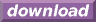#Calcute: freeware calculator

## Cosine (cos)

The cosine of an angle is defined using the right-triangle formed by this angle: it is the ratio of the triangle's adjacent side to its hypotenuse. Calcute implements it with the cos function.

```cos(1)
0.540302305868
```

In the above example, the given angle is in radians.

The triangle involved in the definition of the cosine function is commonly shown in the cartesian plane with its adjacent side on the X axis and its angle point located at the origin (0,0). The other end of the triangle's hypotenuse marks a point on the periphery of a circle centered at the origin; the circle's radius is the length of the hypotenuse. If the angle is increased from 0 to 2 pi radians (0 to 360 degrees) then the end point of the hypotenuse traces a full circle. As the angle of the hypotenuse changes, so does its cosine value as the shape of the corresponding triangle changes to match.

The cosine value remains within the close range [-1, +1].

The cosine function is periodic. It is defined for all real numbers. As angle values increase (or decrease) outside the range of [0, 2 pi] radians that represent one full rotation, the value of cos for these angles repeats itself periodically with each new rotation.